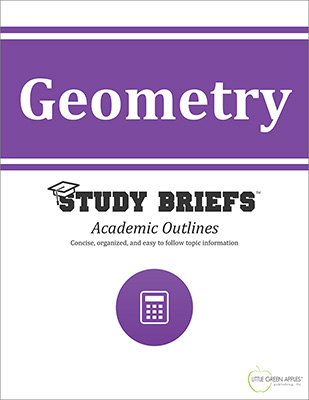# GeometryLook inside
• Format: eBook
• Edition: 2016
• Publisher: Little Green Apples Publishing LLC

Price: \$4.99

## Description:

Got a geometry problem you need to solve quickly? Are you always needing to look up a basic fact or formula? The Geometry Study Brief may be your answer. This quick reference of geometry fundamentals is ideal for students of higher-level math and lifelong learners interested in advanced math topics. This general overview is clear and concise with useful examples of equations presented in a highly visual format. This Study Brief highlights key ideass related to Euclidean Geometry, Plane Geometry, Geometry of Three-Dimensional (3-D) Space, Analytic Geometry, and Non-Euclidean Geometry. Detailed sections focus on these topics: Euclidean postulates, proofs and the Pythagorean Theorem; points, including collinear and non-collinear points; lines, line segments, parallel lines, perpendicular lines, intersecting lines and transversal lines; rays; angles, including types of angles and pairs of angles; polygons, such as circles, triangles, quadrilaterals and three-dimensional shapes, such as cubes, pyramids, prisms, cylinders, and conic sections. Key information on parabolas, ellipses, hyperbolas, congruence, properties, coordinates, ratios and proportions, means and extremes, and transformations is also included.

## Topics Covered:

• History of Geometry
• Key Ideas
• Euclidean Geometry
• Plane Geometry
• Geometry of Three-Dimensional Space
• Analytic Geometry
• Non-Euclidean Geometry
• References
• Online Resources

Browse Subjects